# RS Aggarwal Solutions for Class 10 Maths Chapter 9 Constructions Exercise 9B

Various problems on constructions help students in preparing for the exams in a better way. Get free RS Aggarwal Solutions for Class 10 Maths, Chapter 9 Constructions and get ready for your exams. We have explained steps of constructions using step by step approach with the help of various diagrams. At the end of the section, students will be able to solve any construction problem at their own pace.

## Download PDF of RS Aggarwal Solutions for Class 10 Maths Chapter 9 Constructions Exercise 9B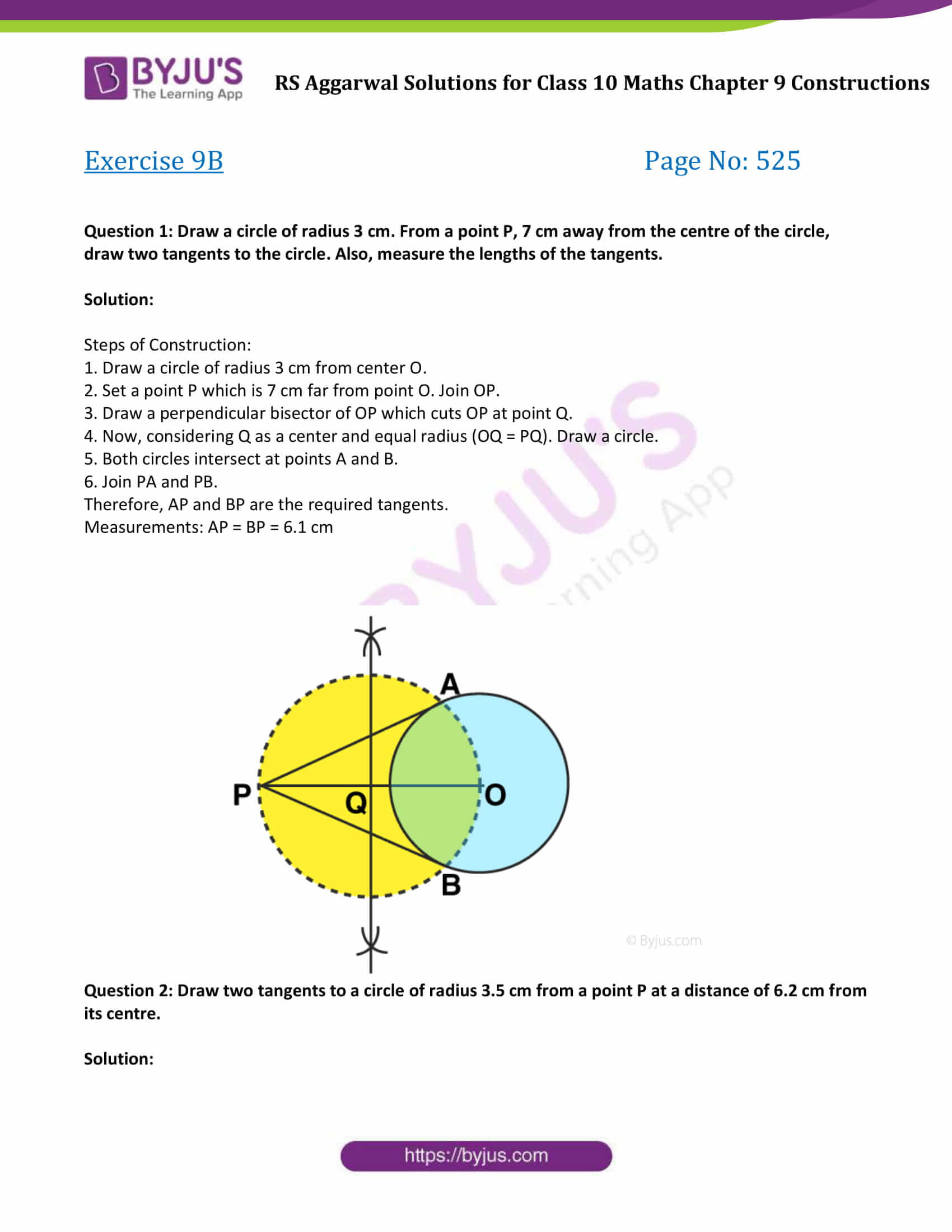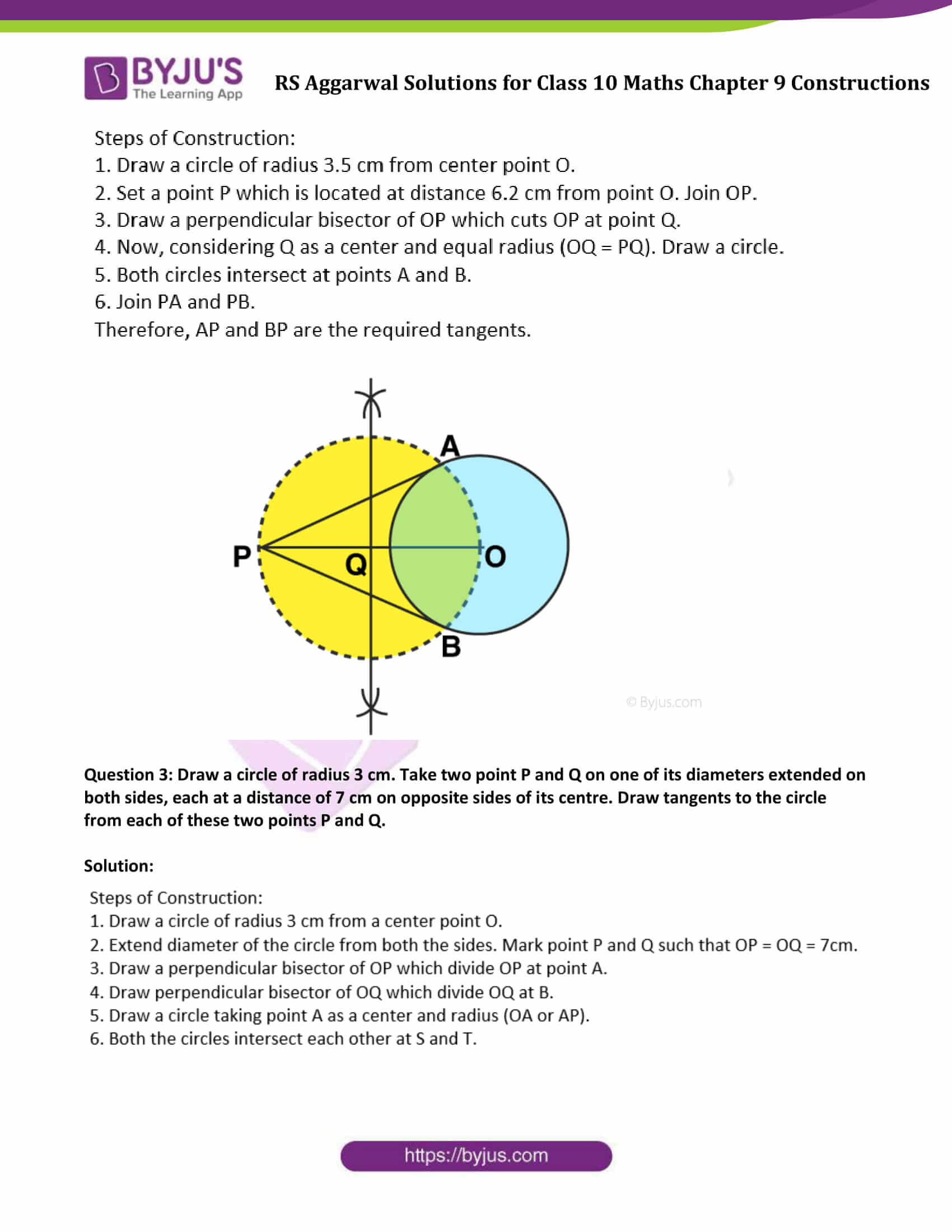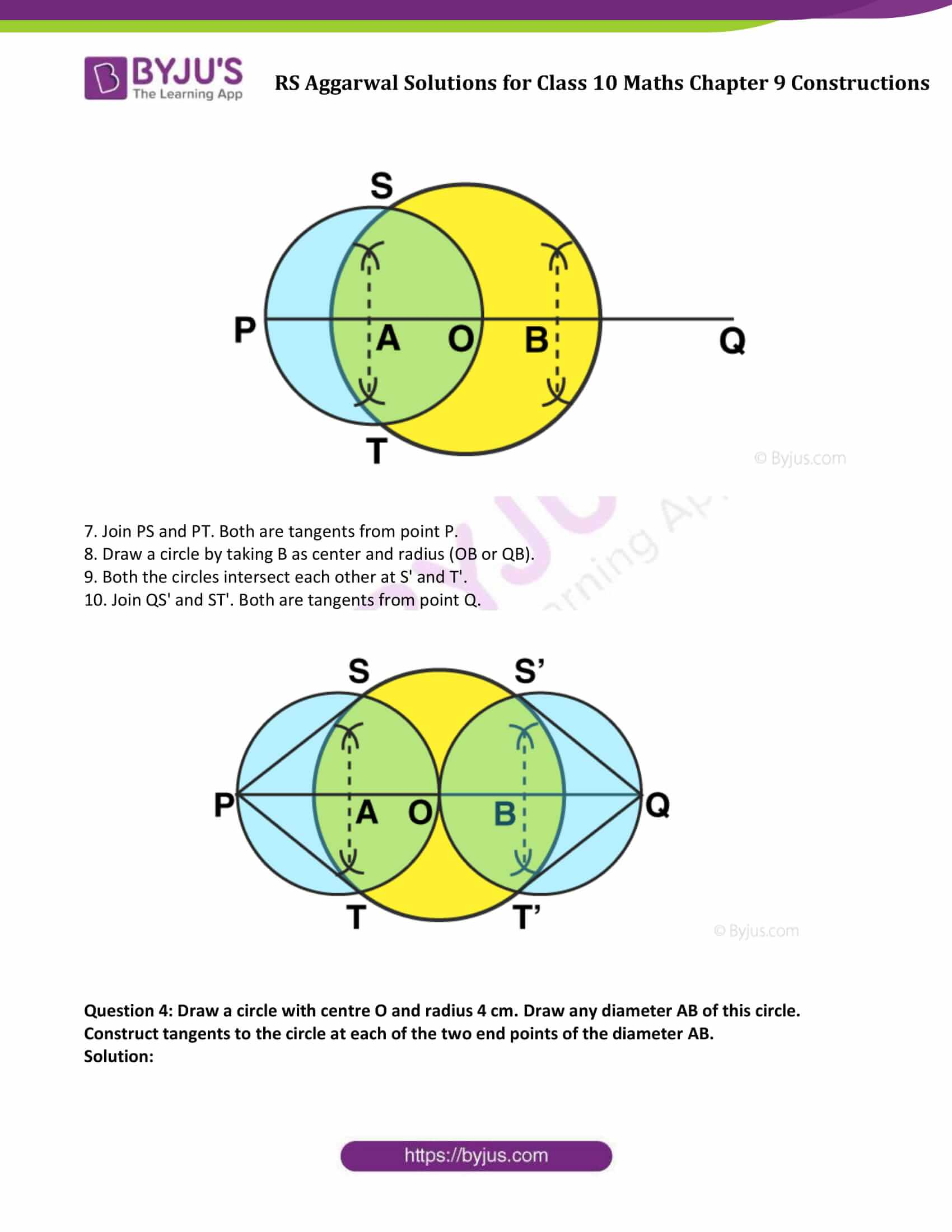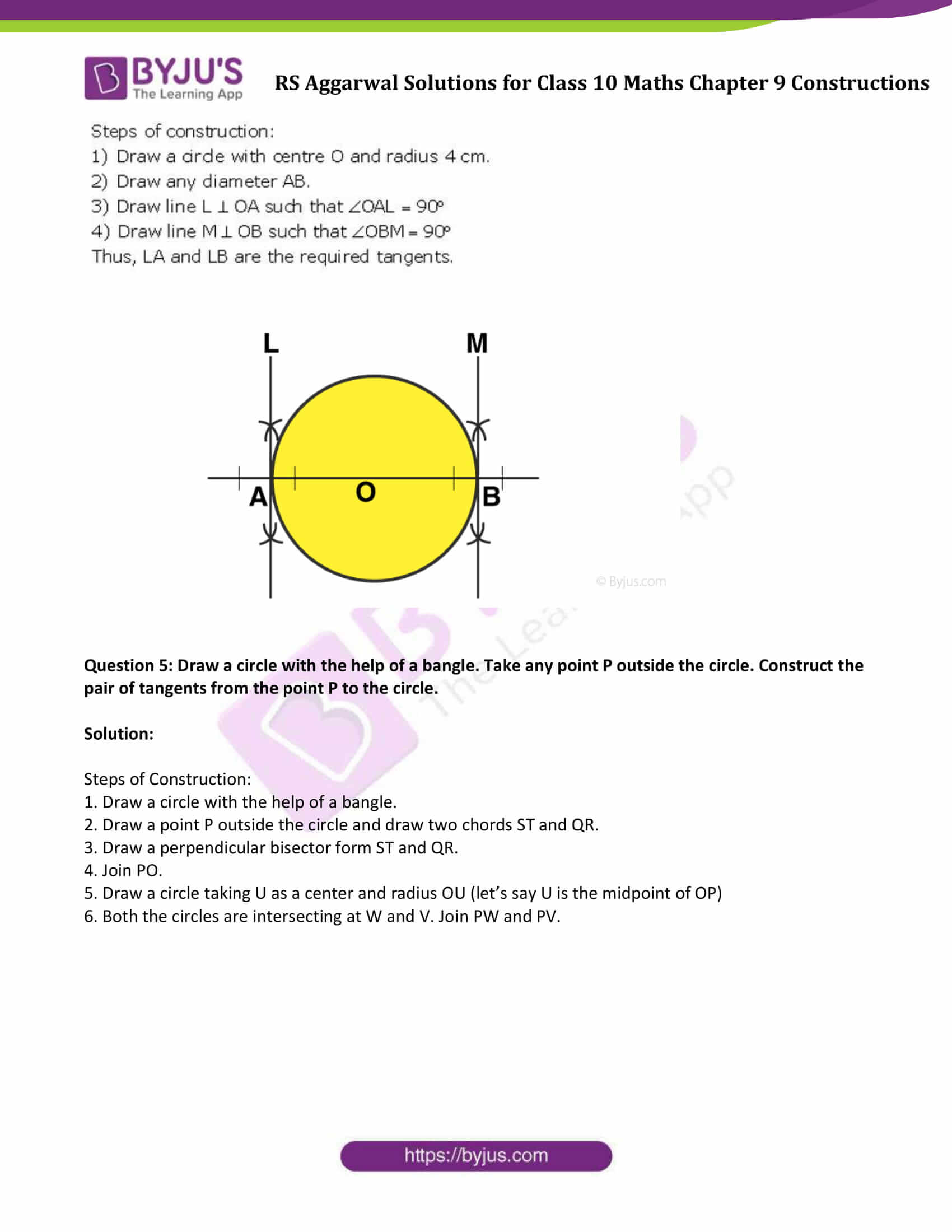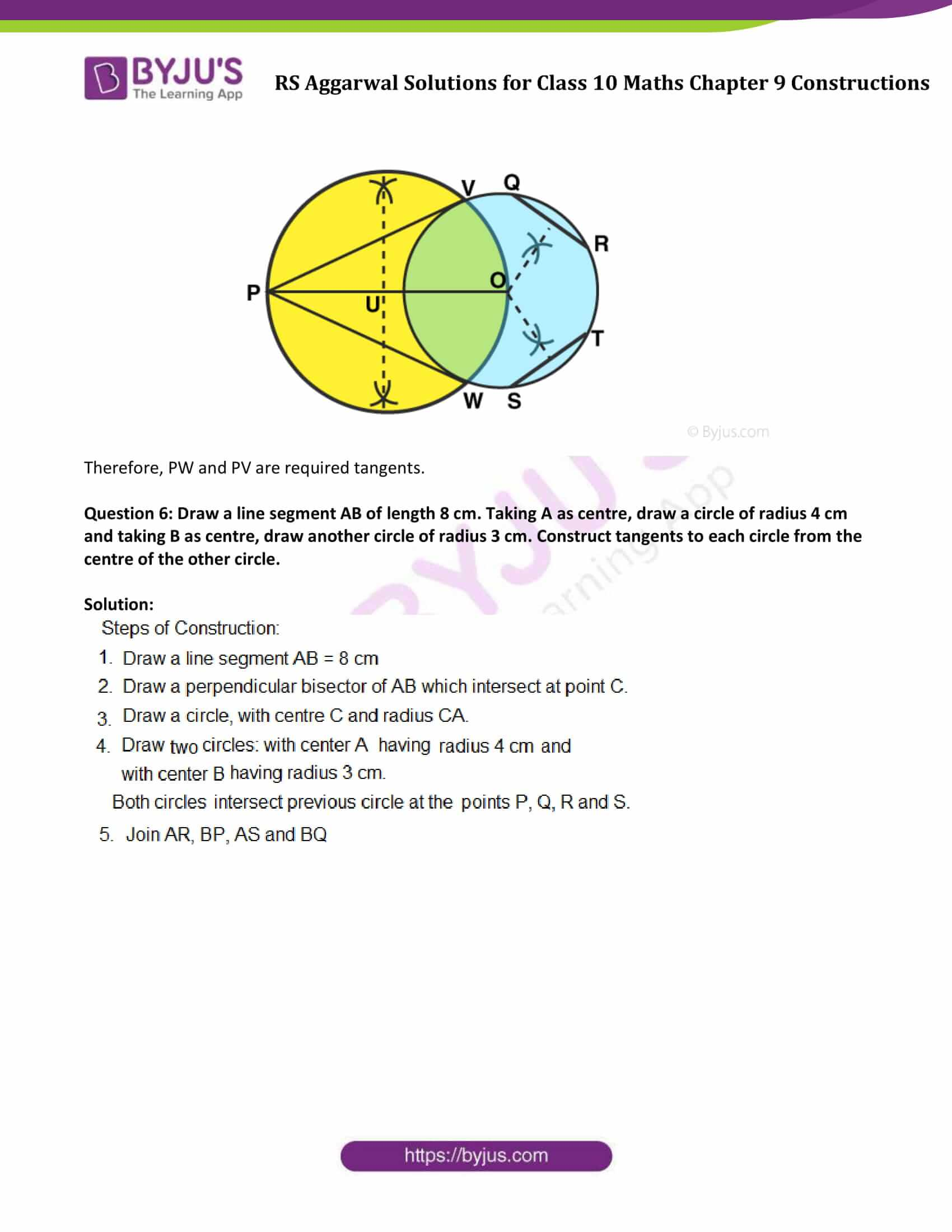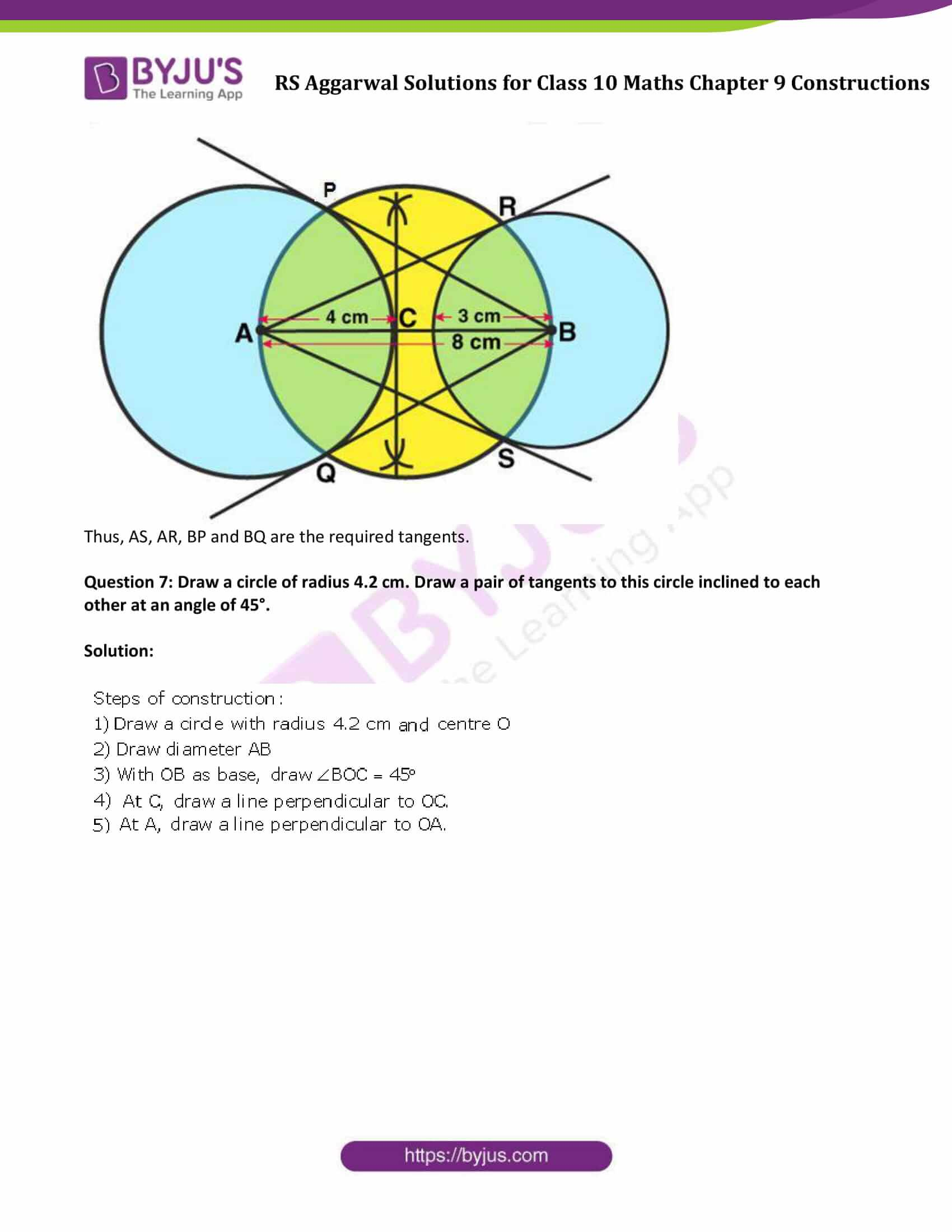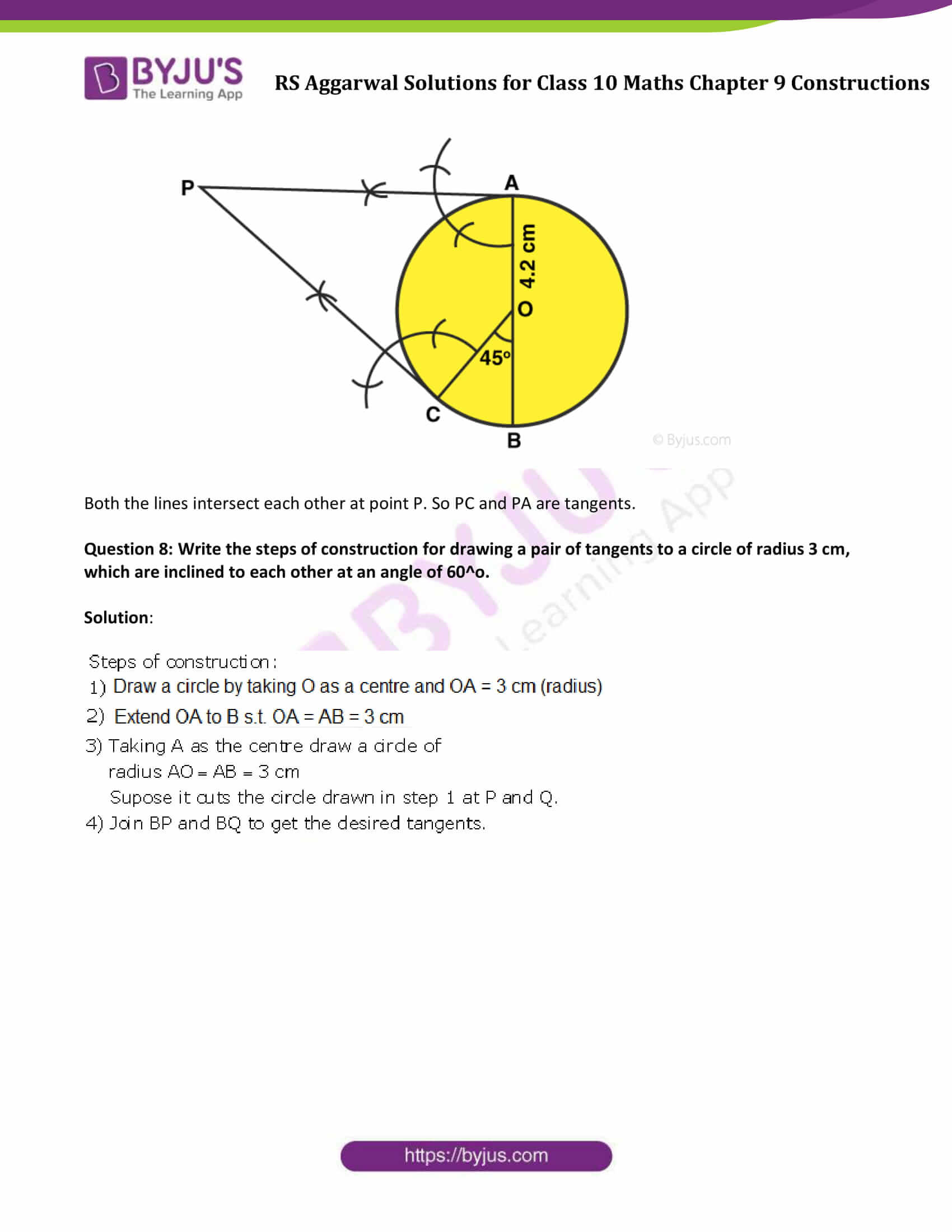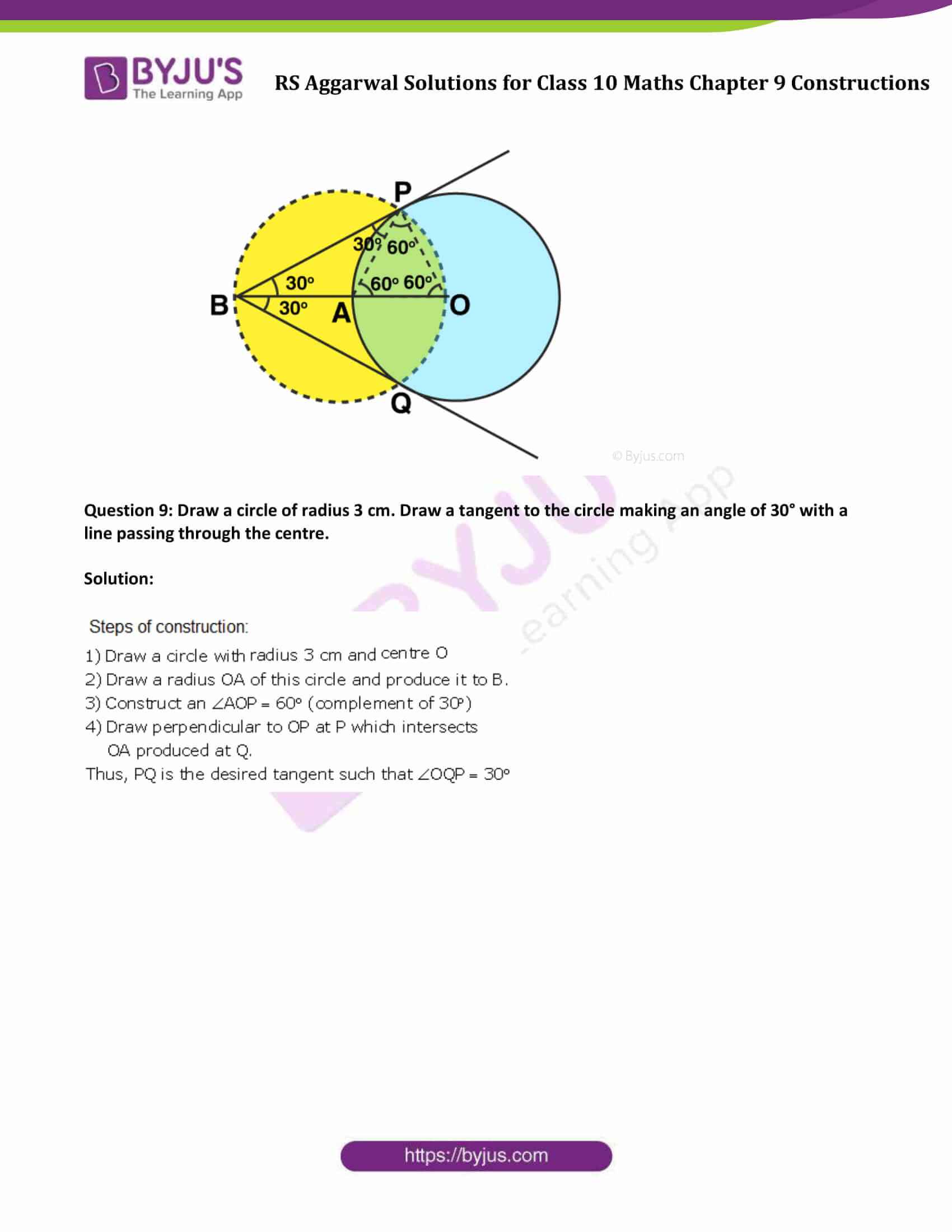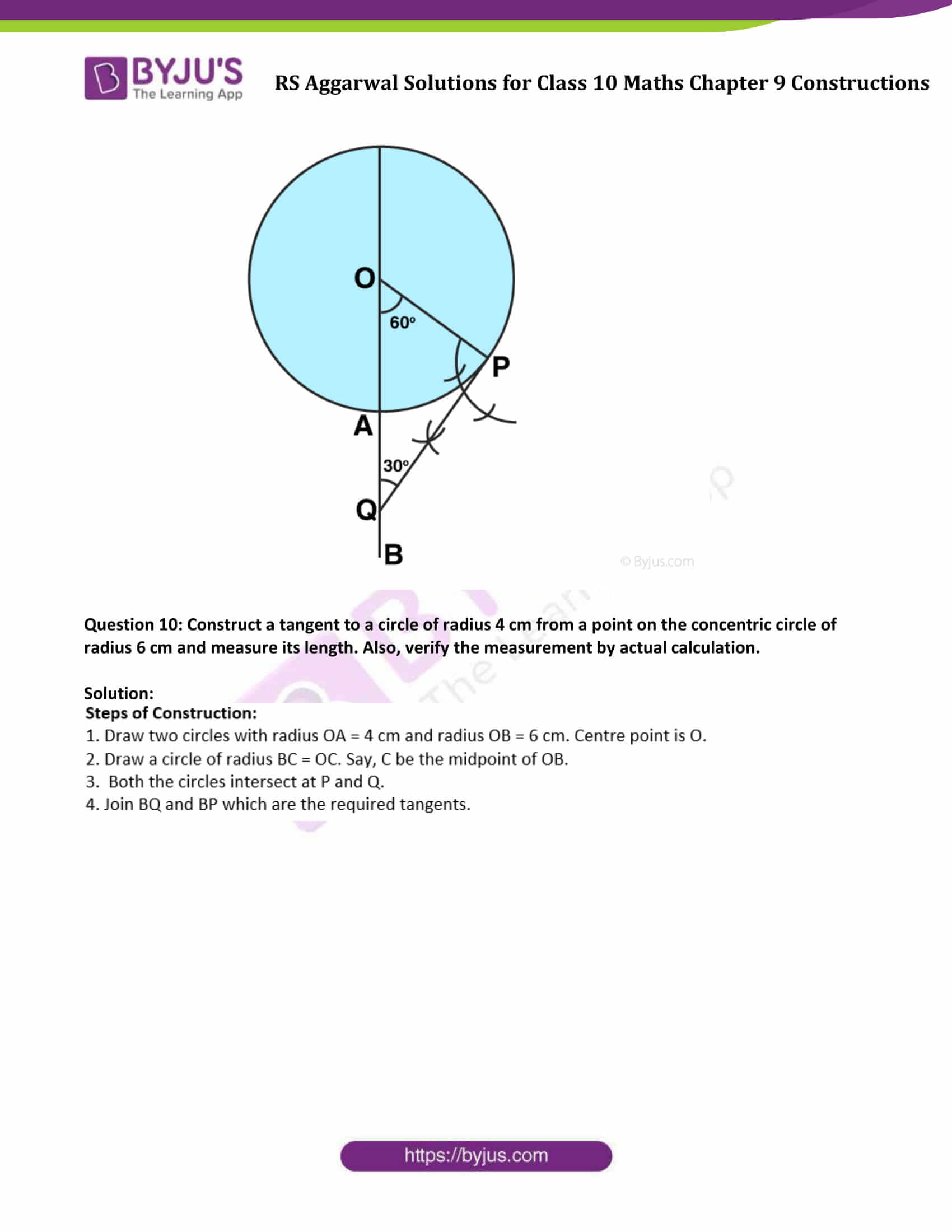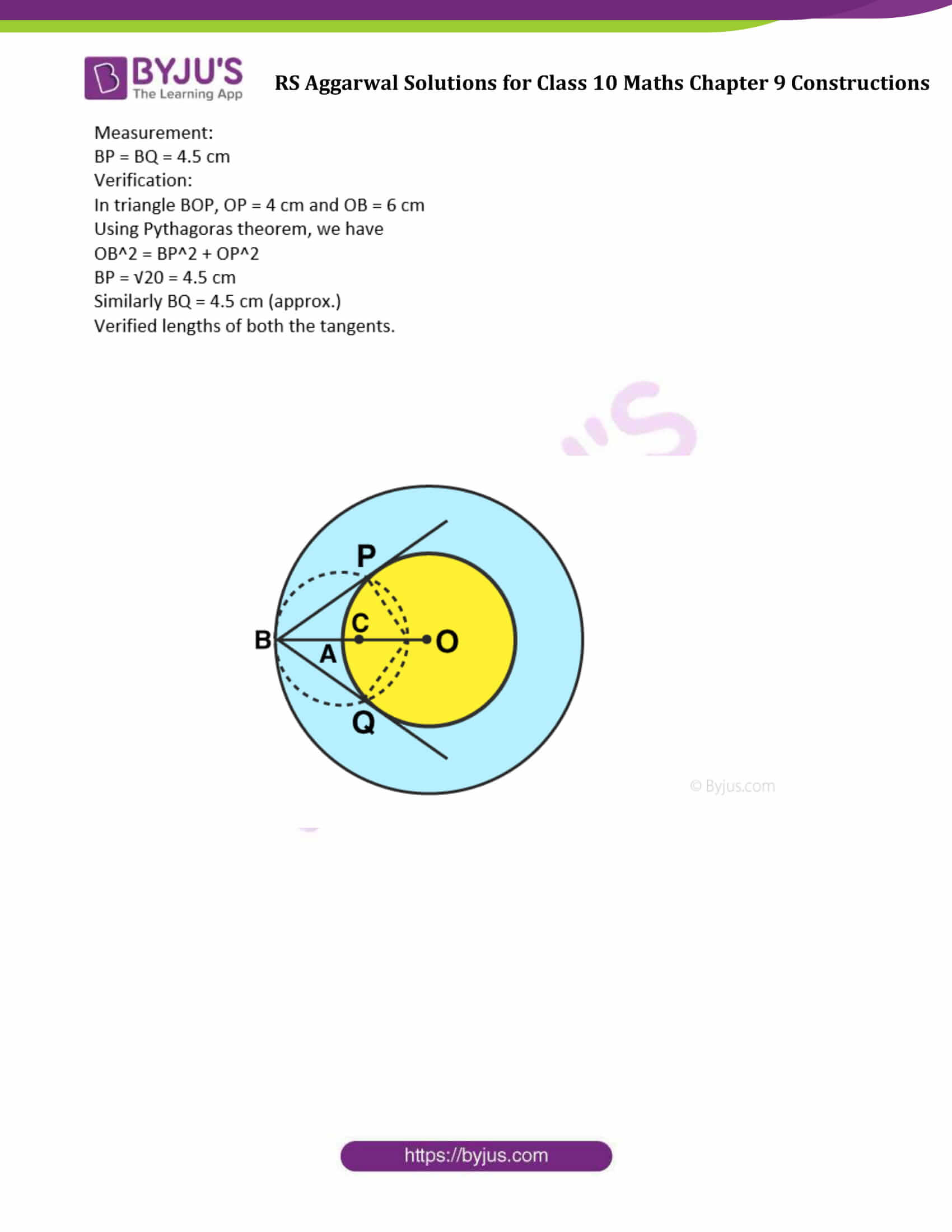### Access other exercise solutions of Class 10 Maths Chapter 9 Constructions

Exercise 9A Solutions

## Exercise 9B

Question 1: Draw a circle of radius 3 cm. From a point P, 7 cm away from the centre of the circle, draw two tangents to the circle. Also, measure the lengths of the tangents.

Solution:

Steps of Construction:

1. Draw a circle of radius 3 cm from center O.

2. Set a point P which is 7 cm far from point O. Join OP.

3. Draw a perpendicular bisector of OP which cuts OP at point Q.

4. Now, considering Q as a center and equal radius (OQ = PQ). Draw a circle.

5. Both circles intersect at points A and B.

6. Join PA and PB.

Therefore, AP and BP are the required tangents.

Measurements: AP = BP = 6.1 cm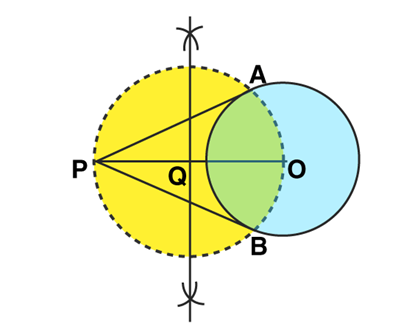Question 2: Draw two tangents to a circle of radius 3.5 cm from a point P at a distance of 6.2 cm from its centre.

Solution: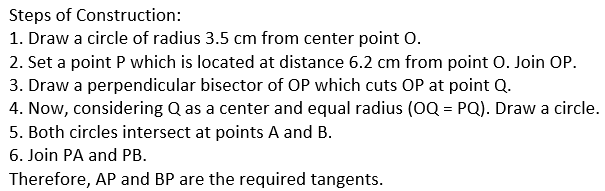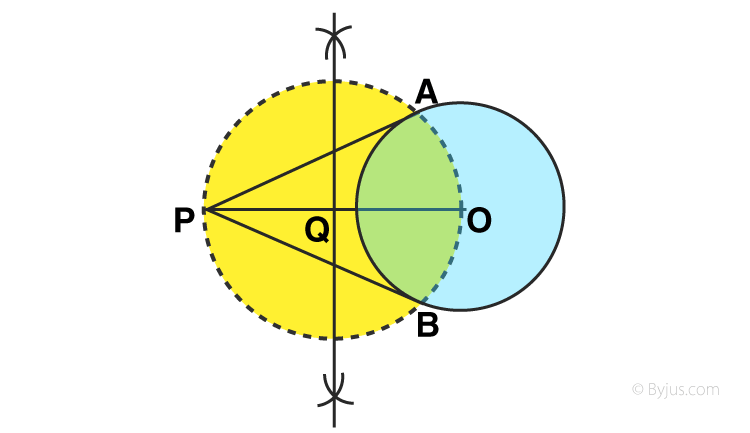Question 3: Draw a circle of radius 3 cm. Take two point P and Q on one of its diameters extended on both sides, each at a distance of 7 cm on opposite sides of its centre. Draw tangents to the circle from each of these two points P and Q.

Solution: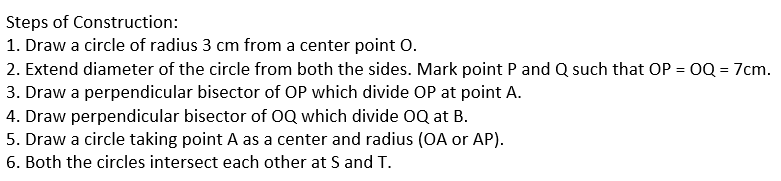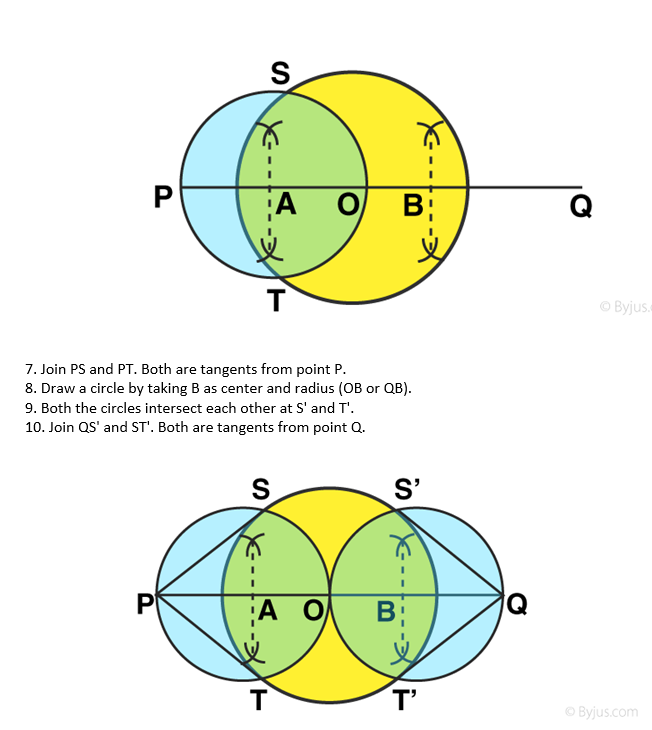Question 4: Draw a circle with centre O and radius 4 cm. Draw any diameter AB of this circle. Construct tangents to the circle at each of the two end points of the diameter AB.

Solution: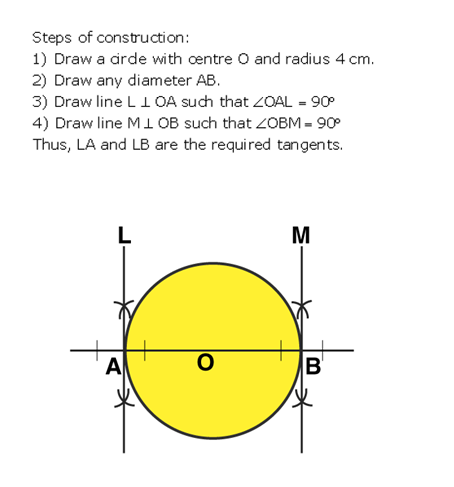Question 5: Draw a circle with the help of a bangle. Take any point P outside the circle. Construct the pair of tangents from the point P to the circle.

Solution:

Steps of Construction:

1. Draw a circle with the help of a bangle.

2. Draw a point P outside the circle and draw two chords ST and QR.

3. Draw a perpendicular bisector form ST and QR.

4. Join PO.

5. Draw a circle taking U as a center and radius OU (let’s say U is the midpoint of OP)

6. Both the circles are intersecting at W and V. Join PW and PV.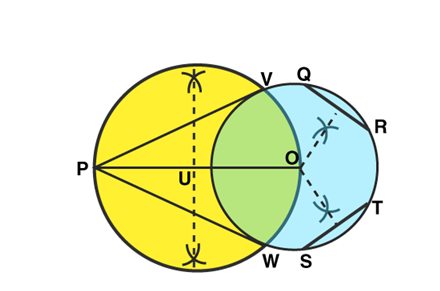Therefore, PW and PV are required tangents.

Question 6: Draw a line segment AB of length 8 cm. Taking A as centre, draw a circle of radius 4 cm and taking B as centre, draw another circle of radius 3 cm. Construct tangents to each circle from the centre of the other circle.

Solution: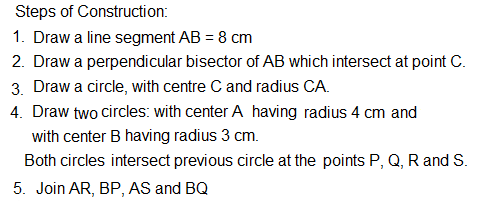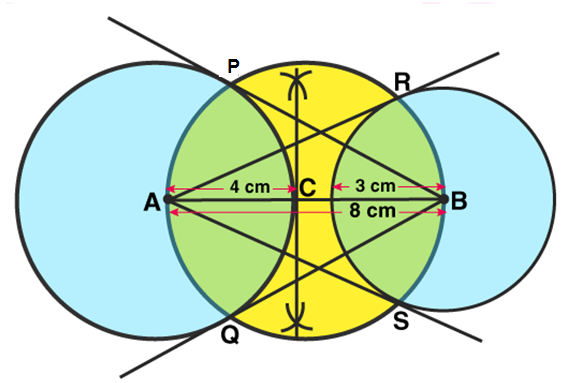Thus, AS, AR, BP and BQ are the required tangents.

Question 7: Draw a circle of radius 4.2 cm. Draw a pair of tangents to this circle inclined to each other at an angle of 45°.

Solution: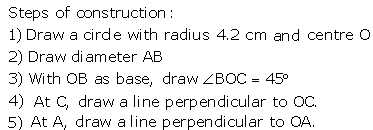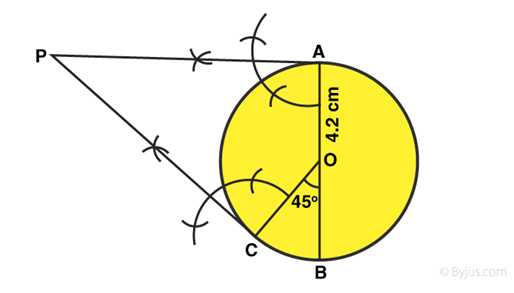Both the lines intersect each other at point P. So PC and PA are tangents.

Question 8: Write the steps of construction for drawing a pair of tangents to a circle of radius 3 cm, which are inclined to each other at an angle of 60^o.

Solution: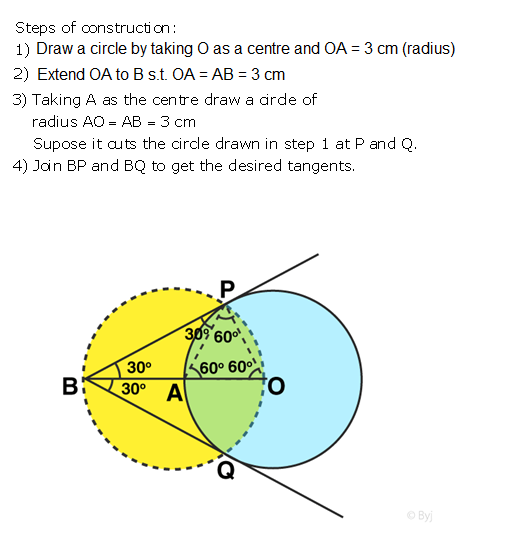Question 9: Draw a circle of radius 3 cm. Draw a tangent to the circle making an angle of 30° with a line passing through the centre.

Solution: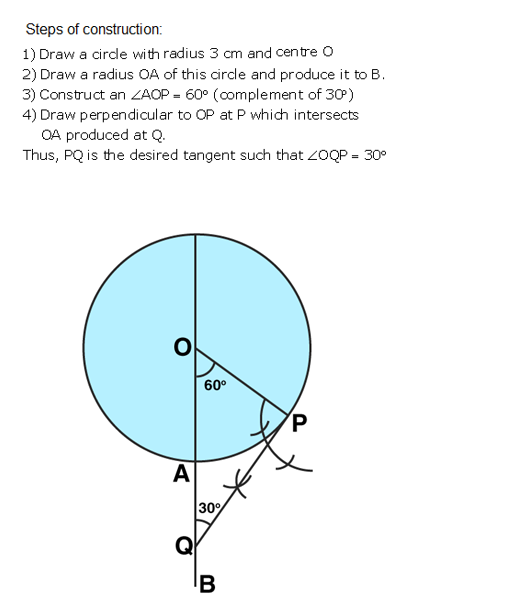Question 10: Construct a tangent to a circle of radius 4 cm from a point on the concentric circle of radius 6 cm and measure its length. Also, verify the measurement by actual calculation.

Solution: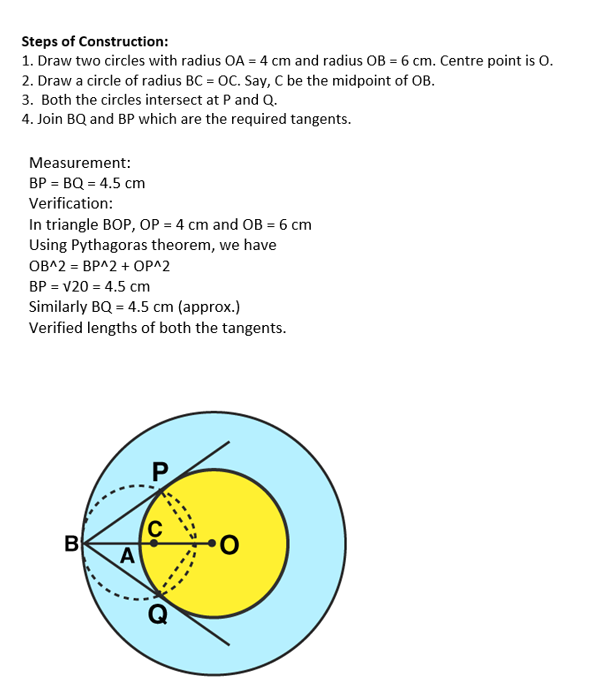## RS Aggarwal Solutions for Class 10 Maths Chapter 9 Constructions Exercise 9B

Class 10 Maths Chapter 9 Constructions Exercise 9B is based on Construction of tangents to a circle. In this exercise, students will get an opportunity to construct

– a tangent to a circle at a given point on the circle

– a tangent to a circle at a point on it without using the centre

– a tangent to a circle from a point outside it when the centre of the circle is known and many more.